# Methods and formulas for conditional statistics in Predict for Fit Mixed Effects Model

Select the method or formula of your choice.

## Conditional fitted value

The conditional fitted values are calculated using the following equation:

### Notation

TermDescription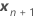the vector of the new settings for the fixed effect terms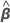the estimated coefficients for the fixed effect terms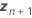The vector of the new settings for the random terms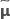he BLUP predictions for the random terms

## Standard error of the conditional fitted value (SE Fit)

The standard error of a conditional fitted value equals the square root of the following variance expression.

where

X is the design matrix for the fixed effect terms and Z is the design matrix for the random terms.

## Confidence intervals for conditional means

The range in which the mean response for a given set of predictor values is expected to fall.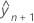is the conditional fit.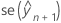is the standard error of he fit.

The degrees of freedom use this formula for the conditional case:

where
and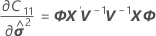### Notation

TermDescription
Wthe asymptotic variance-covariance matrix of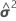For further details on the notation, go to Conditional fits and residuals in Fit Mixed Effects Model.

## Conditional prediction interval

The conditional prediction interval is:is the conditional fit at the new variable setting.==The degrees of freedom use this formula for the conditional case:

where

### Notation

TermDescription
W the asymptotic variance-covariance matrix of the variance component estimates
Ithe identity matrixthe variance component for the ith random effect termthe vector of the new settings for the fixed effect termsthe vector of the new settings for the random terms
cthe number of random terms in the model
BC21
G
mithe number of levels for therandom effect
Xthe n x p design matrix for the fixed effects terms,the inverse of the variance-covariance matrixthe n x mi design matrix for therandom term in the model